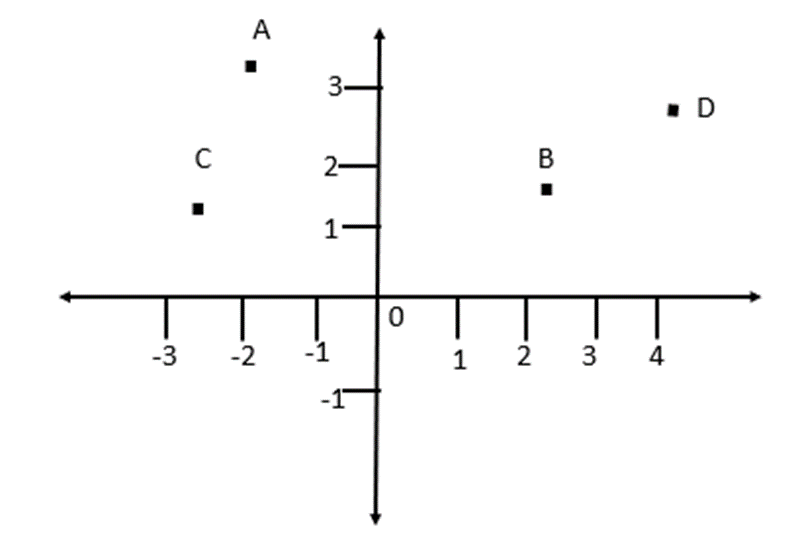Maths-
General
Easy

Question

# Which point in the graph below is (-2,3)## ACDBHint:

## The correct answer is: A

### The four points are A, B, C and DWe have to find the point having coordinates (-2,3)If we compare it with (x,y) we can see that the x coordinate of the given point is -2 and the y coordinate of the point is 3.X coordinate of a point is distance of the point from y-axis and y coordinate of a point is the distance of point from x-axis.From figure we can see that, A is -2 distance from y-axis and it is at distance 3 from x-axis.So, the right option is A.

For such questions, we have to check the distance of point from both the axes.#### With Turito Foundation.#### Get an Expert Advice From Turito.